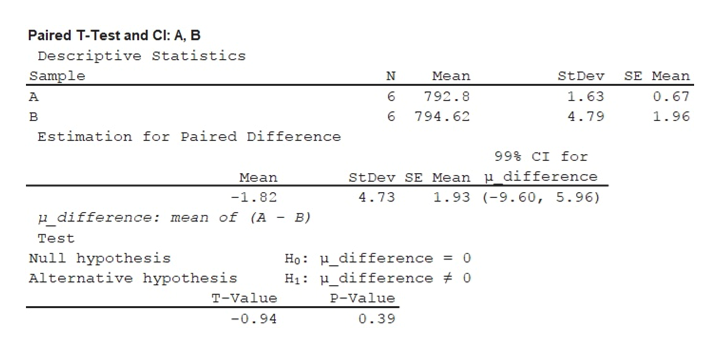# The following data represent the muzzle velocity​ (in feet per​ second) of rounds fired from a​ 155-mm gun. For each​ round, two measurements of the velocity were recorded using two different measuring​ devices, resulting in the following data. Complete parts​ (a) through​ (d) below.Observation123456 A790.2793.3791.6793.9794.7793.1 B794.8789.0796.2793.5802.9791.3 ​(a) Why are these​ matched-pairs data?  A.All the measurements came from rounds fired from the same gun. B.Two measurements​ (A and​ B) are taken on the same round. C.The measurements​ (A and​ B) are taken by the same instrument. D.The same round was fired in every trial.​(b) Is there a difference in the measurement of the muzzle velocity between device A and device B at the α=0.01level of​ significance? ​Note: A normal probability plot and boxplot of the data indicate that the differences are approximately normally distributed with no outliers. Letdiequals=Aiminus−Bi.Identify the null and alternative hypotheses.  H0​:μd=0H1​:μd≠0 Determine the test statistic for this hypothesis test. t0= −0.94​(Round to two decimal places as​ needed.)Find the​ P-value. ​P-value=0.390​(Round to three decimal places as​ needed.) What is your conclusion regardingH0​? Do not reject H0. There is not sufficient evidence at the α=0.01 level of significance to conclude that there is a difference in the measurements of velocity between device A and device B.​(c) Construct a​ 99% confidence interval about the population mean difference. Compute the difference as device A minus device B. Interpret your results. The lower bound is_.The upper bound is_.​(Round to two decimal places as​ needed.)

Question
90 views
The following data represent the muzzle velocity​ (in feet per​ second) of rounds fired from a​ 155-mm gun. For each​ round, two measurements of the velocity were recorded using two different measuring​ devices, resulting in the following data. Complete parts​ (a) through​ (d) below.
 Observation 1 2 3 4 6 5 A 790.2 793.3 791.6 793.9 794.7 793.1 B 794.8 789.0 796.2 793.5 802.9 791.3
​(a) Why are these​ matched-pairs data?

A.
All the measurements came from rounds fired from the same gun.

B.
Two measurements​ (A and​ B) are taken on the same round.

C.
The measurements​ (A and​ B) are taken by the same instrument.

D.
The same round was fired in every trial.
​(b) Is there a difference in the measurement of the muzzle velocity between device A and device B at the α=0.01
level of​ significance? ​Note: A normal probability plot and boxplot of the data indicate that the differences are approximately normally distributed with no outliers.

Let
diequals=Aiminus−Bi.
Identify the null and alternative hypotheses.

H0​:μd=0
H1​:μd≠0

Determine the test statistic for this hypothesis test.

t0= −0.94
​(Round to two decimal places as​ needed.)
Find the​ P-value.

​P-value=0.390
​(Round to three decimal places as​ needed.)

H0​?

Do not reject H0. There is not sufficient evidence at the α=0.01 level of significance to conclude that there is a difference in the measurements of velocity between device A and device B.
​(c) Construct a​ 99% confidence interval about the population mean difference. Compute the difference as device A minus device B. Interpret your results.

The lower bound is_.
The upper bound is_.
​(Round to two decimal places as​ needed.)
check_circle

Step 1

(a) Reason why the data is matched-pairs data:

The data shows that the muzzle velocity of rounds fired from 155-mm gum and two measurements of the velocity were recorded using two different measuring devices.

From the given information, it is observed that the two measurements of A and B are taken on the same round. Thus, the given data represents the matched-pairs data.

The correct option is B. Two measurements (A and B) are taken on the same round.

Step 2

(b) Testing whether there is a difference in the measurement of the muzzle velocity between device A and device B:

The hypotheses are given below:

Null hypothesis:

H0: µd = 0

Alternative hypothesis:

Ha: µd ≠ 0

Test statistic:

Step-by-step procedure to obtain the test statistic and P-value using the MINITAB software:

• Choose Stat > Basic Statistics > Paired t.
• In FirstSample, enter the column of A.
• In Second Sample, enter the column of B.
• Check Options, enter Confidence level as 0.
• Choose Not equal in alternative.
• Click OK in all dialogue boxes.

Output using the MINITAB software is given below:help_outlineImage TranscriptionclosePaired T-Test and Cl: A, B Descriptive Statistics Sample N Mean StDev SE Mean 0.67 6 792.8 1.63 A. 6 794.62 4.79 1.96 в Estimation for Paired Difference 99 CI for StDev SE Mean p_difference Mean -1.82 4.73 1.93 9.60, 5.96) u difference: mean of (A - B) Test Ho: H_difference = 0 Hi H_difference 0 Null hypothesis Alternative hypothesis T-Value P-Value -0.94 0.39 fullscreen
Step 3

From the MINITAB output, the test statistic is −0.94 and the P- value is 0.39.

Decision rule:

If P-value ≤ α, reject the null hypothesis.

If P-value > α, fail to reject the null hypothesis.

Conclusion:

From the output, it is observed that the P-value is 0.39.

Here, P-value is greater than the level of significance.

That is, P-value (=0.39) > α&n...

### Want to see the full answer?

See Solution

#### Want to see this answer and more?

Solutions are written by subject experts who are available 24/7. Questions are typically answered within 1 hour.*

See Solution
*Response times may vary by subject and question.
Tagged in

### Other